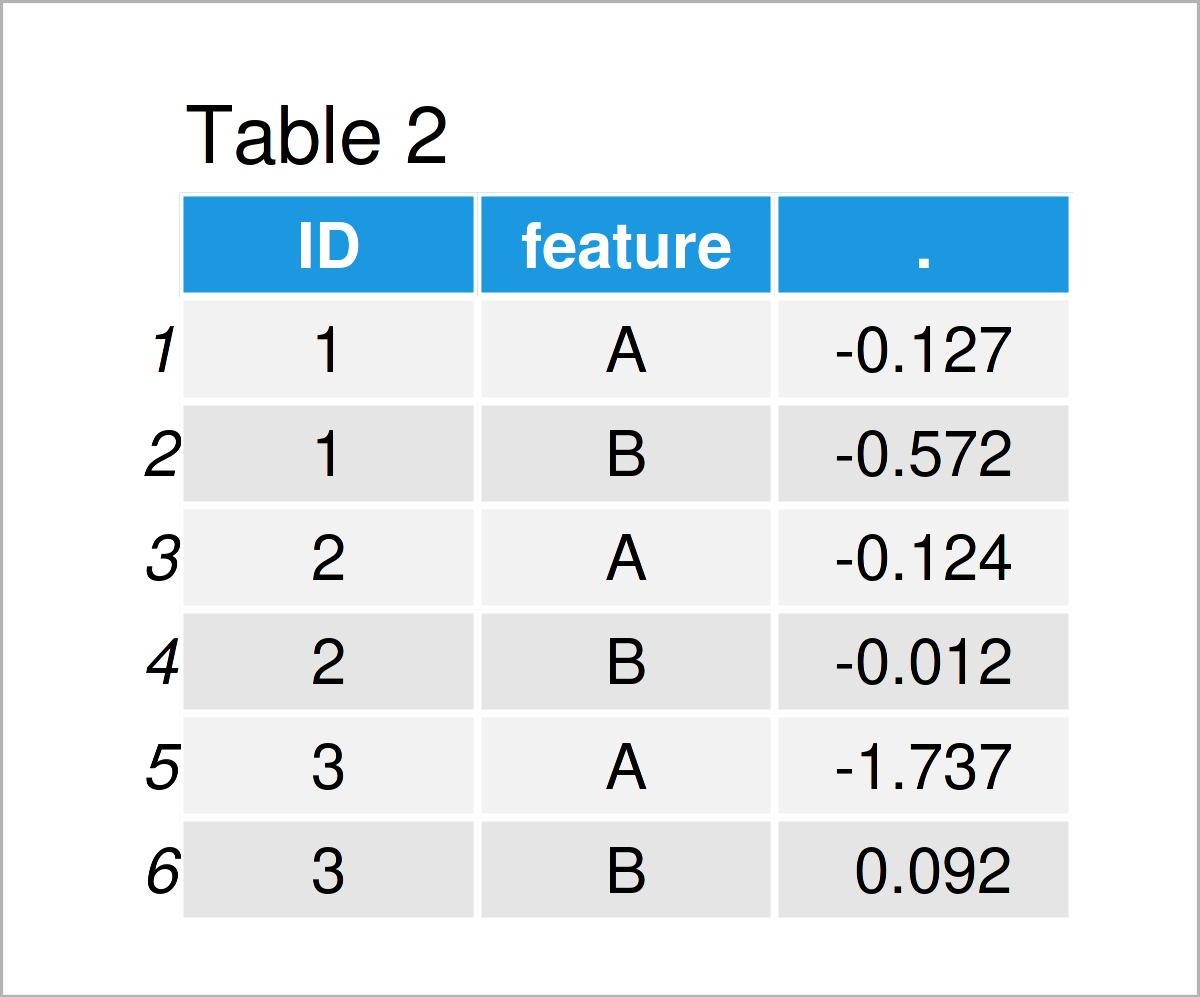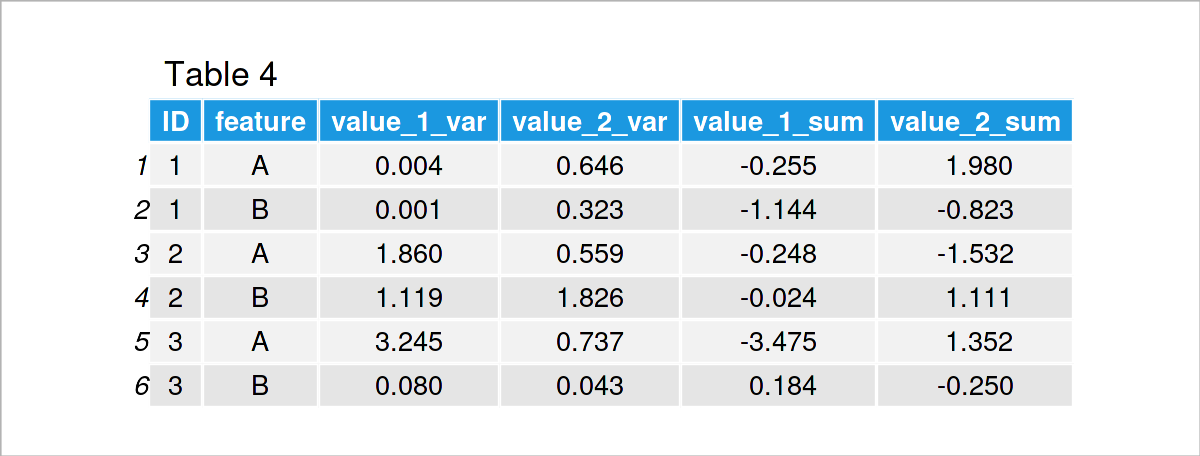# dcast data.table Function in R (3 Examples)

In this R tutorial you’ll learn how to reshape a data.table, for example summarizing the data for specific groups or reordering the rows according to specific features.

The page is structured as follows:

Let’s do this…

## Example Data & Software Packages

First, we need to install and load the data.table package:

```install.packages("data.table") # Install data.table package

In addition, have a look at the exemplifying data below.

```set.seed(8)
combinations <- expand.grid(ID      = 1:3,
feature = c("A", "B"),
time    = 1:2)
DT_1 <- setDT(cbind(combinations,
"value_1" =  rnorm(nrow(combinations)),
"value_2" =  rnorm(nrow(combinations))))Table 1 reveals the head of our example data – Furthermore, you can see that our data consists of five variables. With the function expand.grid(), we generated some synthetic experimental data, where for each ID (for example a person) we have features A and B observed in time point 1 and 2 with two values value_1 and value_2.

## Example 1: Group Means

Example 1 demonstrates how to use the dcast() function of the data.table package to calculate statistics of the data.

```DT_2 <- dcast(DT_1,
ID + feature ~ .,
fun.aggregate = mean,
value.var     = "value_1")
head(DT_2)                     # Counts of observations for each combination```Table 2 shows the output of the previous syntax: Using the data.table DT_1, we calculated the mean value of the numeric variable value_1 for the combinations of ID and feature.

## Example 2: Multiple Functions

In Example 2, I’ll illustrate how to apply multiple functions in the dcast() function.

```DT_3 <- dcast(DT_1,
ID + feature ~ .,
fun.aggregate = list(mean, sum),
value.var     = "value_1")By executing the previous R syntax, we have created Table 3, i.e. we not only calculated the mean value, but also the sum of the numeric variable value_1 for the combinations of ID and feature.

## Example 3: Multiple Variables with Numeric Values

Example 3 illustrates how to calculate multiple statistics for multiple numeric variables of a data.table object with the dcast() function.

```DT_4 <- dcast(DT_1,
ID + feature ~ .,
fun.aggregate = list(var, sum),
value.var     = c("value_1", "value_2"))In Table 4 you can see that we have managed to construct a data.table which contains the variance and the sum of variables value_1 and value_2 for the combinations of ID and feature.

## Example 4: Reshape the Data

In this example, we use the dcast() function to reshape our data. That is, we can use dcast() to order our data rows according to specific variables.

```DT_5 <- dcast(DT_1,
ID + time + feature ~ .,
value.var = c("value_1", "value_2"))Table 5 reveals the output of the previous R programming syntax – Before, the data was ordered as time, feature, ID. Now it is ordered as ID, time, feature.

## Video, Further Resources & Summary

Do you want to learn more about the reshaping of a data.table with dcast? Then I can recommend watching the following data.table video on my YouTube channel. I’m explaining the R code of this tutorial in the video.

In addition, you could have a look at the related tutorials on my website.

To summarize: On this page, you have learned how to handle the dcast long-to-wide reshaping tool for data.tables in the R programming language. Please let me know in the comments section, in case you have further questions.

This page was created in collaboration with Anna-Lena Wölwer. Have a look at Anna-Lena’s author page to get further details about her academic background and the other articles she has written for Statistics Globe.

Subscribe to the Statistics Globe Newsletter

•Roos de Gouw
December 6, 2022 12:04 pm

Hello,
I found it very confusing that you only showed the data for time 1 and not for time 2.
I didn’t know that the head() function only gives you the first part of a vector.
Because of this it is impossible to calculate yourself the mean for the ID and feature as shown in table 2 and thus understanding what the dcast function does.
Only in table 5 you show the data for time 2 and that is how you could get the mean as shown in table 2.

I suggest you show all the original data in the beginning so people can calculate the mean themselfs as well and so understand what is happening in the function.

•December 13, 2022 2:14 pm